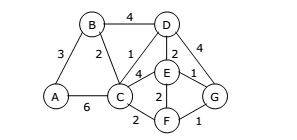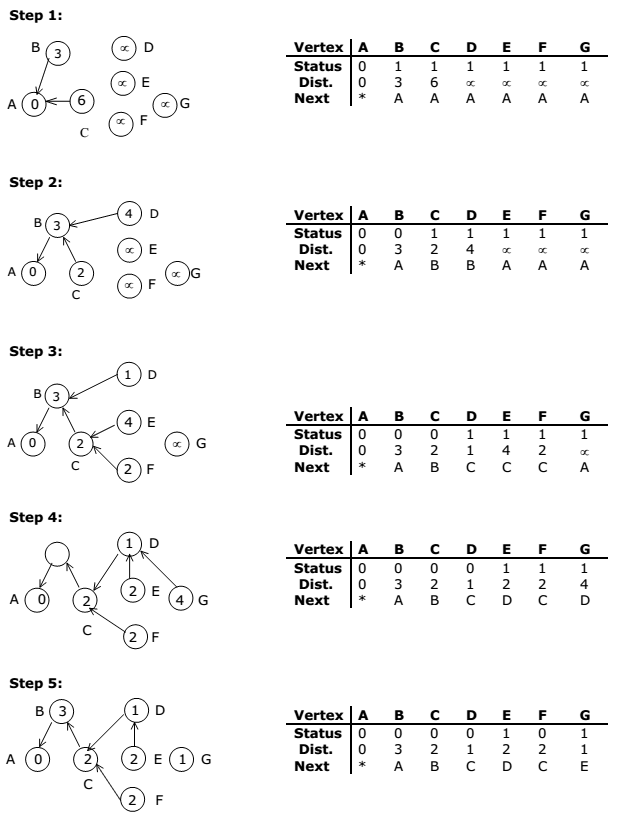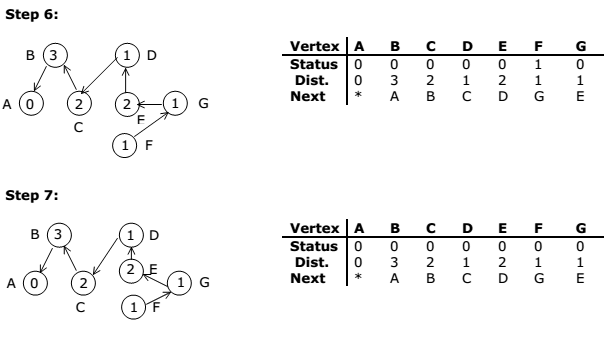# Prim’s Algorithm-minimal cost spanning tree A+ A  A-

A given graph can have many spanning trees. From these many spanning trees, we
have to select a cheapest one. This tree is called as minimal cost spanning tree.

Minimal cost spanning tree is a connected undirected graph G in which each edge is
labeled with a number (edge labels may signify lengths, weights other than costs).
Minimal cost spanning tree is a spanning tree for which the sum of the edge labels is as
small as possible

The slight modification of the spanning tree algorithm yields a very simple algorithm for
finding an MST. In the spanning tree algorithm, any vertex not in the tree but
connected to it by an edge can be added. To find a Minimal cost spanning tree, we
must be selective – we must always add a new vertex for which the cost of the new
edge is as small as possible.

This simple modified algorithm of spanning tree is called prim’s algorithm for finding an
Minimal cost spanning tree. Prim’s algorithm is an example of a greedy algorithm.

Prim’s Algorithm:
E is the set of edges in G. cost [1:n, 1:n] is the cost adjacency matrix of an n vertex
graph such that cost [i, j] is either a positive real number or ∝ if no edge (i, j) exists. A
minimum spanning tree is computed and stored as a set of edges in the array t [1:n-1,
1:2]. (t [i, 1], t [i, 2]) is an edge in the minimum-cost spanning tree. The final cost is
returned.

EXAMPLE:
Use Prim’s Algorithm to find a minimal spanning tree for the graph shown below
starting with the vertex A.Solution:The stepwise progress of the prim’s algorithm is as follows: